```(:mini m9=90x45 m8=50x75 m7=40x40 m6=0x60:) Horizontal thumbs 90x45 pixels (with Mini9 m9=90x45) : mini9:* Vertical thumbs 50x75 pixels (with Mini8 m8=50x75) : mini8:* Square thumbs 40x40 pixels (with Mini7 m7=40x40) : mini7:* Fixed height 60px, proportional width (Mini6 m6=0x60) : mini6:*``` Horizontal thumbs 90x45 pixels (with Mini9 m9=90x45) :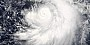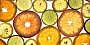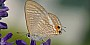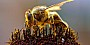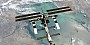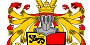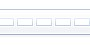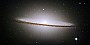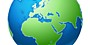Vertical thumbs 50x75 pixels (with Mini8 m8=50x75) :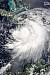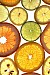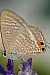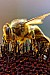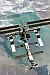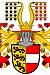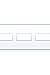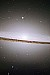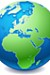Square thumbs 40x40 pixels (with Mini7 m7=40x40) :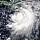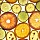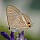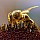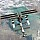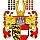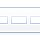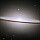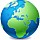Fixed height 60px, proportional width (Mini6 m6=0x60) :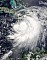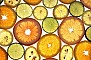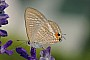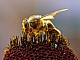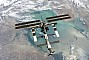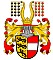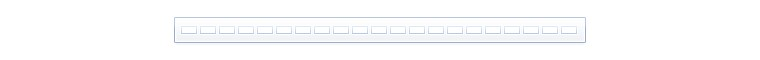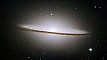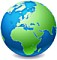In the above examples, lowercase `mini9:` is used, and as expected, it produces no links. If it were capitalized `Mini9:`, there would be links and a slideshow.